# 1557 Happy Birthday to My Brother, Andy!

### Today’s puzzle:

Today is my brother Andy’s birthday. He enjoys solving puzzles so I made this 18 × 18 puzzle hoping that he will find it challenging.This 18 × 18 multiplication table will be invaluable as you work to solve it. When you look for a clue in the table, its color will let you know how many times it appears in the table.Print the puzzles or type the solution in this excel file: 10 Factors 1546-1557.

If you need a hint to solve the puzzle: One of the first things you will want to do is identify the clues that are multiples of 5, 10, or 15. Then use logic to determine which clues will use the two 5’s, the two 10’s, and the two 15’s.

### Factors of 1557:

• 1557 is a composite number.
• Prime factorization: 1557 = 3 × 3 × 173, which can be written 1557 = 3² × 173.
• 1557 has at least one exponent greater than 1 in its prime factorization so √1557 can be simplified. Taking the factor pair from the factor pair table below with the largest square number factor, we get √1557 = (√9)(√173) = 3√173.
• The exponents in the prime factorization are 2 and 1. Adding one to each exponent and multiplying we get (2 + 1)(1 + 1) = 3 × 2 = 6. Therefore 1557 has exactly 6 factors.
• The factors of 1557 are outlined with their factor pair partners in the graphic below.### More about the number 1557:

From OEIS.org we learn that 1557 has a rather fun square:
1557² = 2424249.

1557 is the sum of two squares:
39² + 6² = 1557.

1557 is the hypotenuse of a Pythagorean triple:
468-1485-1557, which is 9 times (52-165-173).
It can also be calculated from 2(39)(6), 39² – 6², 39² + 6².

1557 is also the difference of two squares three different ways:
779² – 778² = 1557,
261² – 258² = 1557, and
91² – 82² = 1557.

# 1555 Two Turkeys Too Tough To Try?

### Today’s Puzzle:

Two turkeys too tough to try? That’s a six-word title made with alliteration and three homophones! It also describes the mystery-level turkey puzzles below. Those turkeys might look like identical twins at first glance, but if you look closely, you will see they are not quite the same.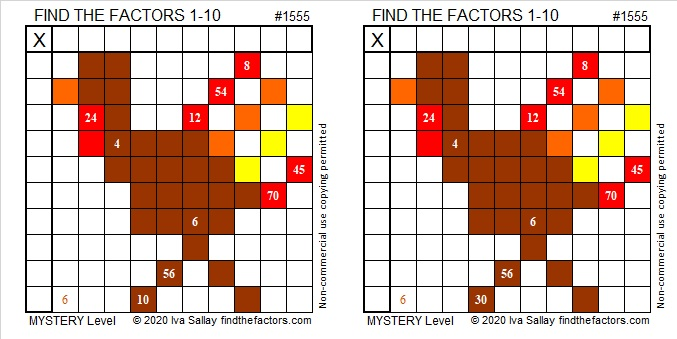Here are some questions to help you find a logical way to start either puzzle: Which two clues MUST use the two 6’s as factors? Are there any other clues that are multiples of 6? If so, what factors would those clues use?

### Factors of 1555:

• 1555 is a composite number.
• Prime factorization: 1555 = 5 × 311.
• 1555 has no exponents greater than 1 in its prime factorization, so √1555 cannot be simplified.
• The exponents in the prime factorization are 1 and 1. Adding one to each exponent and multiplying we get (1 + 1)(1 + 1) = 2 × 2 = 4. Therefore 1555 has exactly 4 factors.
• The factors of 1555 are outlined with their factor pair partners in the graphic below.### Another Fact about the Number 1555:

1555 is the hypotenuse of a Pythagorean triple:
933-1244-1555, which is (3-4-5) times 311.

# 1544 Final Letter of the Message

### Today’s Puzzle:

This is the sixth and final letter of the message that I made for you. It’s a mystery puzzle, but that doesn’t necessarily mean that’s it’s a difficult puzzle. Give it a try and think about why I sent the message.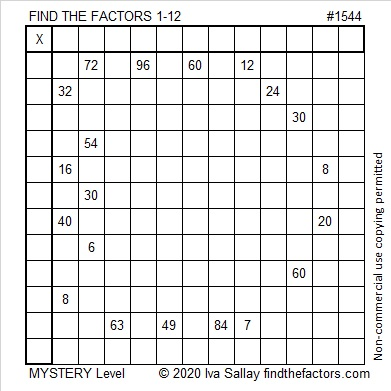### Factors of 1544:

• 1544 is a composite number.
• Prime factorization: 1544 = 2 × 2 × 2 × 193, which can be written 1544 = 2³ × 193.
• 1544 has at least one exponent greater than 1 in its prime factorization so √1544 can be simplified. Taking the factor pair from the factor pair table below with the largest square number factor, we get √1544 = (√4)(√386) = 2√386.
• The exponents in the prime factorization are 3 and 1. Adding one to each exponent and multiplying we get (3 + 1)(1 + 1) = 4 × 2 = 8. Therefore 1544 has exactly 8 factors.
• The factors of 1544 are outlined with their factor pair partners in the graphic below.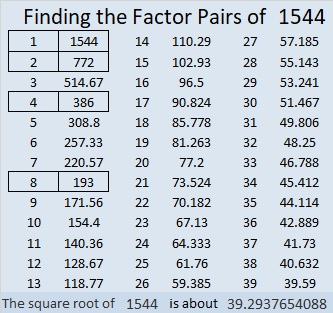### More Facts about the Number 1544:

1544 is the sum of two squares:
38² + 10² = 1544.

1544 is the hypotenuse of a Pythagorean triple:
760-1344-1544, calculated from 2(38)(10), 38² – 10², 38² + 10².
It is also 8 times (95-168-193).

# 1539 Mystery Puzzle for a Mystery Message

### Today’s Puzzle:

This is the third letter in my message to you. Have you figured out the complete message yet?

The puzzle itself is a mystery level puzzle. Will it be easy or difficult to do? You’ll have to try it to know the answer to that one!### Factors of 1539:

Adding up the non-nine digits we get 1 + 5 + 3 = 9, so 1539 is divisible by 9. In fact, it is divisible by 9 twice!

• 1539 is a composite number.
• Prime factorization: 1539 = 3 × 3 × 3 × 3 × 19, which can be written 1539 = 3⁴ × 19.
• 1539 has at least one exponent greater than 1 in its prime factorization so √1539 can be simplified. Taking the factor pair from the factor pair table below with the largest square number factor, we get √1539 = (√81)(√19) = 9√19.
• The exponents in the prime factorization are 4 and 1. Adding one to each exponent and multiplying we get (4 + 1)(1 + 1) = 5 × 2 = 10. Therefore 1539 has exactly 10 factors.
• The factors of 1539 are outlined with their factor pair partners in the graphic below.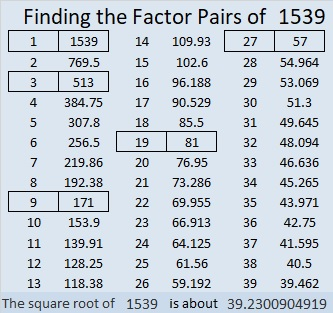### More about the Number 1539:

1539 is the difference of two squares in five different ways:
770² – 769² = 1539,
258² – 255² = 1539,
90² – 81² = 1539,
50² – 31² = 1539, and
42² – 15² = 1539.

# 1533 Double Double Toil and Trouble

### Today’s Puzzle:

Solving this cauldron-looking mystery level puzzle might be double-double toil and trouble.  Why? There are four sets of clues that have more than one possible common factor. Nevertheless, the puzzle has only one solution, and you can find it if you let logic and a basic multiplication table be your guide.### Factors of 1533:

Double Double Toil and Trouble… You can use two divisibility tricks to find the factors of 1533, and the second trick uses doubling.

Is 1533 divisible by 3? The trick for finding numbers divisible by 3 is to add up their digits. If the sum is divisible by 3, so is the original number:
1 + 5 + 3 + 3 = 12, so 1533 is divisible by 3.
It really is! See what I mean: 1533 ÷ 3 = 511.

Is it divisible by 7? Look at the previous quotient, 511. Separate the last digit from the rest and subtract its double from the first part: 51 – 2 = 49, so 511 is divisible by 7, and its multiple, 1533, is also divisible by 7.

(We could have used the same doubling trick TWICE on 1533, but that definitely would have been double-double, toil, and trouble. Just dividing 1533 by 7 to get 219 would be easier!)

• 1533 is a composite number.
• Prime factorization: 1533 = 3 × 7 × 73.
• 1533 has no exponents greater than 1 in its prime factorization, so √1533 cannot be simplified.
• The exponents in the prime factorization are 1, 1, and 1. Adding one to each exponent and multiplying we get (1 + 1)(1 + 1)(1 + 1) = 2 × 2 × 2 = 8. Therefore 1533 has exactly 8 factors.
• The factors of 1533 are outlined with their factor pair partners in the graphic below.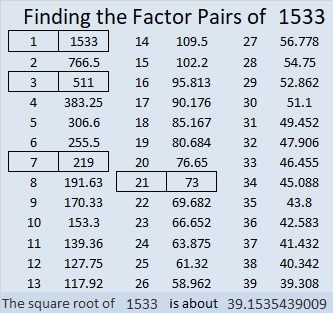### More Facts About the Number 1533:

1533 is the hypotenuse of a Pythagorean triple, and you can apply those same two divisibility tricks to all three numbers in that triple. (Note: 3 × 7 = 21)
1008-1155-1533 which is 21 times (48-55-73)

1533 is the difference of two squares in FOUR different ways:
767² – 766² = 1533,
257² – 254² = 1533,
113² – 106² = 1533, and
47² – 26² = 1533.

# 1524 Mystery

### Today’s Puzzle:

Is this puzzle easy or difficult? That is the mystery. Will any of the clues trick you into writing factors that won’t work with the rest of the puzzle? If you consistently use logic and not just multiplication and division facts, you’ll solve this puzzle.### Factors of 1524:

• 1524 is a composite number.
• Prime factorization: 1524 = 2 × 2 × 3 × 127, which can be written 1524 = 2² × 3 × 127.
• 1524 has at least one exponent greater than 1 in its prime factorization so √1524 can be simplified. Taking the factor pair from the factor pair table below with the largest square number factor, we get √1524 = (√4)(√381) = 2√381.
• The exponents in the prime factorization are 2, 1, and 1. Adding one to each exponent and multiplying we get (2 + 1)(1 + 1)(1 + 1) = 3 × 2 × 2 = 12. Therefore 1524 has exactly 12 factors.
• The factors of 1524 are outlined with their factor pair partners in the graphic below.### More about the Number 1524:

1524 is the difference of two squares in two different ways:
382² – 380² = 1524,
130² – 124² = 1524.

# 1523 Mystery Puzzle

### Today’s Puzzle:

My newly turned 10-year-old granddaughter likes making Find the Factors 1 -12 Puzzles with me. Unfortunately, at the beginning of the month, she was in an accident. Her 12-year-old cousin hadn’t ever made a puzzle before but helped me make this one to wish her a speedy recovery. (Thankfully, she is almost fully recovered now.)### Factors of 1523:

• 1523 is a prime number.
• Prime factorization: 1523 is prime.
• 1523 has no exponents greater than 1 in its prime factorization, so √1523 cannot be simplified.
• The exponent in the prime factorization is 1. Adding one to that exponent we get (1 + 1) = 2. Therefore 1523 has exactly 2 factors.
• The factors of 1523 are outlined with their factor pair partners in the graphic below.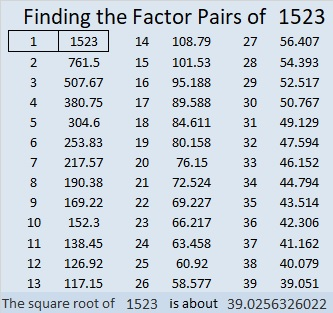How do we know that 1523 is a prime number? If 1523 were not a prime number, then it would be divisible by at least one prime number less than or equal to √1523. Since 1523 cannot be divided evenly by 2, 3, 5, 7, 11, 13, 17, 19, 23, 29, 31, or 37, we know that 1523 is a prime number.

### Another Fact about the Number 1523:

1523 is the difference of two consecutive squares:
762² – 761² = 1523.

# 1522 Happy Birthday, Sue, in Spite of All the Chaos!

### Today’s Puzzle:

Today is my sister’s birthday. This year has been a turbulent and chaotic year for many people including her. A hurricane completely damaged her home this summer. Sue, here’s hoping that the coming year will be much brighter for you. Here is a chaotic-looking puzzle for your birthday. If you find all the products after you find all the factors, it will look a lot more orderly. Have a very happy birthday today!### Factors of 1522:

• 1522 is a composite number.
• Prime factorization: 1522 = 2 × 761.
• 1522 has no exponents greater than 1 in its prime factorization, so √1522 cannot be simplified.
• The exponents in the prime factorization are 1, and 1. Adding one to each exponent and multiplying we get (1 + 1)(1 + 1) = 2 × 2 = 4. Therefore 1522 has exactly 4 factors.
• The factors of 1522 are outlined with their factor pair partners in the graphic below.### More Facts about the Number 1522:

1522 is the sum of two squares:
39² + 1² = 1522.

1522 is the hypotenuse of a Pythagorean triple:
78-1520-1522 calculated from 2(39)(1), 39² – 1² , 39² + 1².

From OEIS.org we learn this curious fact:
The digits of 1522 are 1, 5, 2, and 2.
The squares of each of those digits are 1, 25, 4, and 4.
12544 is a perfect square. It is 112².

# 1509 A Mystery Level Puzzle on Training Wheels

### Today’s Puzzle:

I have described level 3 puzzles as level 4 puzzles on training wheels. Today’s puzzle is definitely not a level 3 puzzle, but it is on training wheels. A logical way to solve this puzzle is to start with the clue in the top row and work your way down the puzzle row by row writing the factors as you go. Giving you the logical order to use the clues should help some, but the logic needed to find the factors will still be a mystery. Don’t guess and check. Please, use logic! Think about how each clue relates to the other clues in the puzzle.### Factors of 1509:

• 1509 is a composite number.
• Prime factorization: 1509 = 3 × 503.
• 1509 has no exponents greater than 1 in its prime factorization, so √1509 cannot be simplified.
• The exponents in the prime factorization are 1, and 1. Adding one to each exponent and multiplying we get (1 + 1)(1 + 1) = 2 × 2 = 4. Therefore 1509 has exactly 4 factors.
• The factors of 1509 are outlined with their factor pair partners in the graphic below.### More about the Number 1509:

1509 is the difference of two squares in two different ways:
755² – 754² = 1509
253² – 250² = 1509

# 1508 Hosting a Playful Math Carnival and Flying by the Seat of My Pants

### Blog Submission Appeal:

Please, tell me how you’ve made K-12 math education more fun. You see, later this month I’m hosting the Playful Math Education Blog Carnival. I have found several great blog posts to share, but maybe I haven’t seen yours. You can share your blog post with me by submitting this official form, leaving a comment below, or messaging me on twitter, Iva Sallay@findthefactors.com. I look forward to reading your post! Please share it with me by Saturday, August 22 so it can be included in this month’s carnival.

### Today’s Puzzle:

This mystery-level puzzle was modeled after a carnival ride, the swing carousel, a ride that tilts slightly as it goes around, and lets you ride by the seat of your pants. My puzzle might not be the best representation of that ride, but it hopefully got your attention.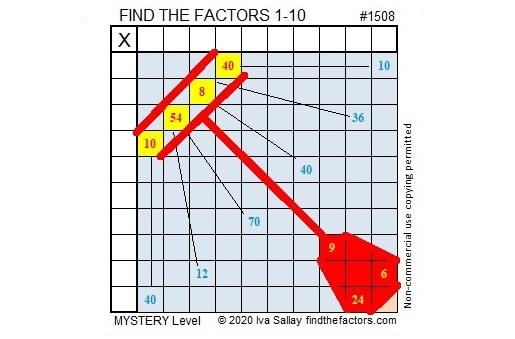Embellishing the puzzle might make it more eye-catching, but it is probably easier to solve the puzzle without distracting color and lines. (It’s a mystery-level puzzle, so I’m keeping how easy or difficult it is a secret.) Here is a plain version of the same puzzle: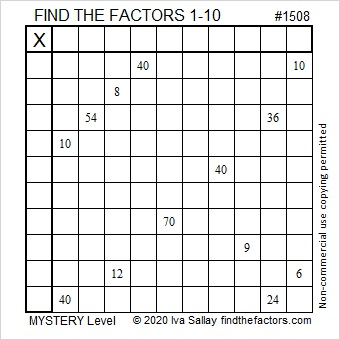### Factors of 1508:

• 1508 is a composite number.
• Prime factorization: 1508 = 2 × 2 × 13 × 29, which can be written 1508 = 2² × 13 × 29.
• 1508 has at least one exponent greater than 1 in its prime factorization so √1508 can be simplified. Taking the factor pair from the factor pair table below with the largest square number factor, we get √1508 = (√4)(√377) = 2√377.
• The exponents in the prime factorization are 2, 1, and 1. Adding one to each exponent and multiplying we get (2 + 1)(1 + 1)(1 + 1) = 3 × 2 × 2 = 12. Therefore 1508 has exactly 12 factors.
• The factors of 1508 are outlined with their factor pair partners in the graphic below.### More about the Number 1508:

1508 is the sum of two squares in two different ways:
32² + 22² = 1508
38² + 8² = 1508

1508 is the hypotenuse of FOUR Pythagorean triples:
540-1408-1508, calculated from 32² – 22², 2(32)(22), 32² + 22²,
580-1392-1508, which is (5-12-13) times 116,
608-1380-1508, calculated from 2(38)(8), 38² – 8², 38² + 8²,
1040-1092-1508 which is (20-21-29) times 52.